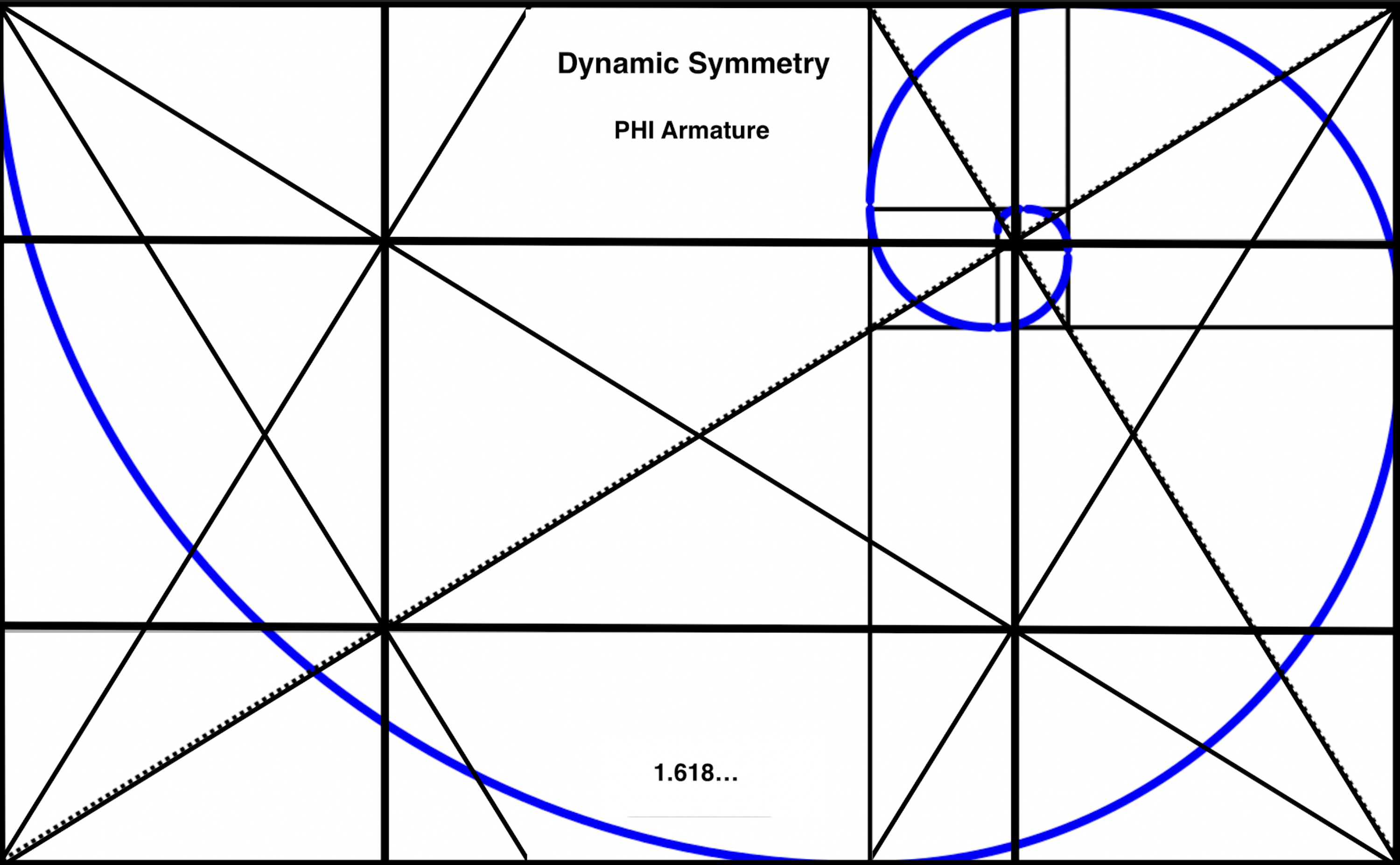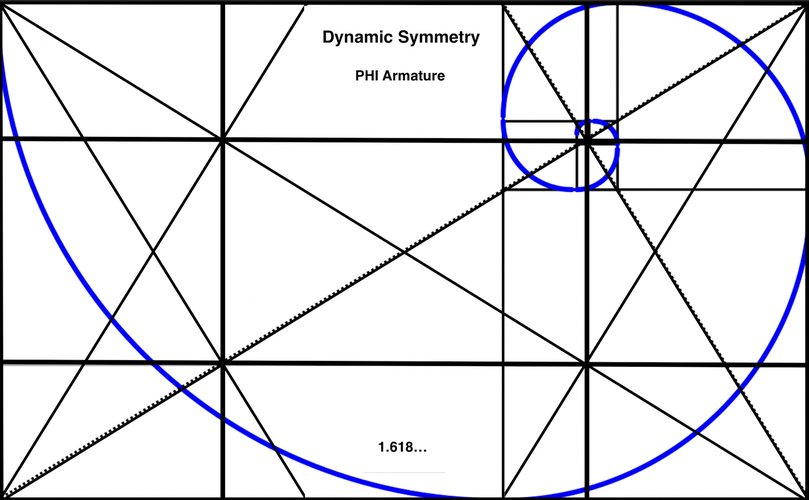## PHI Dynamic Symmetry Armature with a Golden Spiral Approximation

Material
Oil
Size
PHI Aspect Ratio

Expanded+ Armature for our "Golden" paintings (or near-golden), superimposing the spiral onto the Expanded Dynamic Symmetry Armature. The golden spiral is approximated by the Fibonacci or Whirling Squares' Spiral. The whirling squares are constructed within rectangles as they rotate around a point which is sometimes denoted the eye of the spiral (in this case at the upper right). Note in passing that the squares formed here have side lengths, successive Fibonacci terms: 0,1,1,2,3,5,8,13 (in reverse), etc, where each term is the sum of the previous two terms. Nice Diagram Here.
The ratios of successive Fibonacci terms gets closer and closer to the Golden Ratio (1.168...): 2/1 = 2, 3/2 = 1.5, 5/3 - 1.666.., 8/5 = 1.6, 13/8=1.625, 21/13=1.615..., 34/21=1.619..., 55/34=1.6176..,